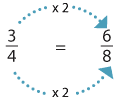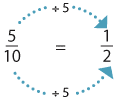### Multiplying and dividing to get equivalent fractions

We have seen that $$\dfrac{3}{4} \text{and} \dfrac{6}{8}$$ are equivalent. You might have noticed that we can multiply the numerator and the denominator of $$\dfrac{3}{4}$$ by the same number to get $$\dfrac{6}{8}$$.Detailed description

Dividing the numerator and denominator by the same whole number also results in an equivalent fraction.Detailed description

So we get an equivalent fraction with a related denominator. Fractions with related denominators are easy to work with when adding and subtracting fractions.